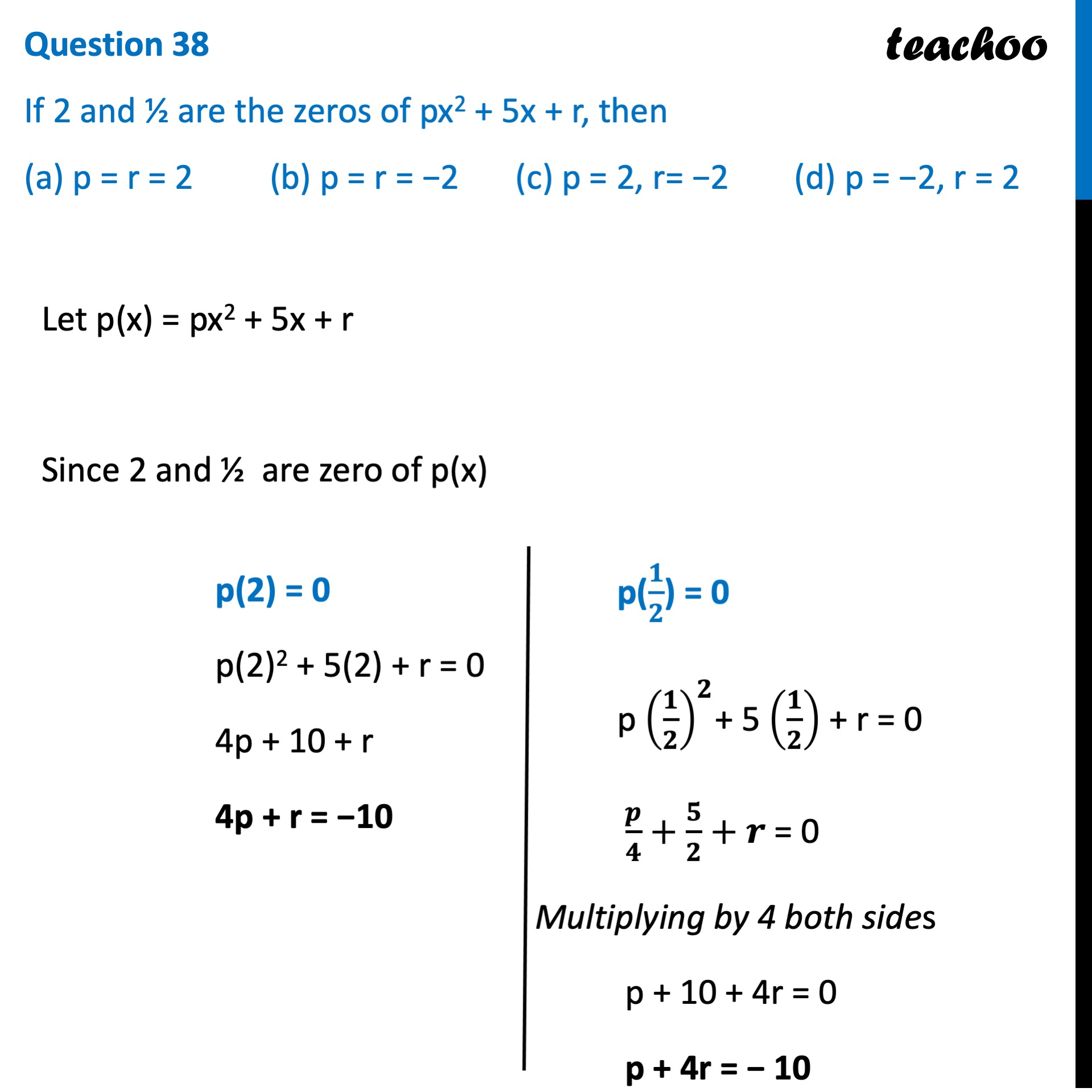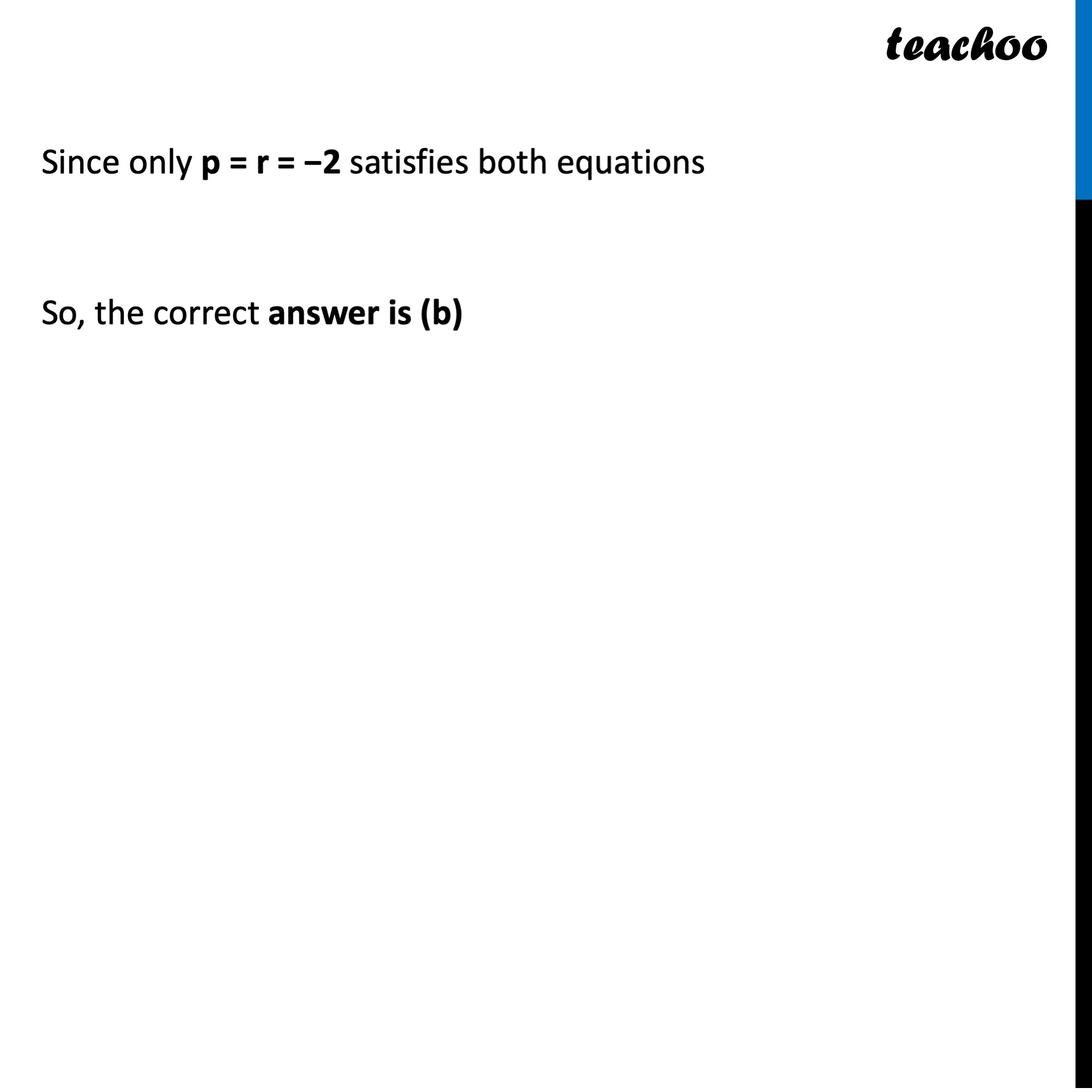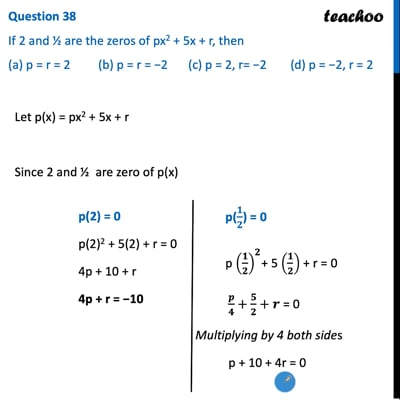CBSE Class 10 Sample Paper for 2022 Boards - Maths Standard [MCQ]

Class 10
Solutions of Sample Papers for Class 10 Boards

## (a) p = r = 2   (b) p = r = −2   (c) p = 2, r= −2       (d) p = −2, r = 2This video is only available for Teachoo black users

Introducing your new favourite teacher - Teachoo Black, at only ₹83 per month

### Transcript

Question 38 If 2 and ½ are the zeros of px 2+5x+r, then (a) p = r = 2 (b) p = r = −2 (c) p = 2, r= −2 (d) p = −2, r = 2 Let p(x) = px2 + 5x + r Since 2 and ½ are zero of p(x) p(2) = 0 p(2)2 + 5(2) + r = 0 4p + 10 + r 4p + r = −10 p(𝟏/𝟐) = 0 p (𝟏/𝟐)^𝟐+ 5 (𝟏/𝟐) + r = 0 𝒑/𝟒+𝟓/𝟐+𝒓 = 0 Multiplying by 4 both sides p + 10 + 4r = 0 p + 4r = − 10 Since only p = r = −2 satisfies both equations So, the correct answer is (b)